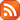Subscribe by RSS

# Prairie Mathematics Colloquium

Thu., Mar. 10, 2022 2:30 p.m.

Location: Live Stream

Time:  Social tea break at 2:00 p.m., Talk at 2:30 p.m.

Speaker: Karen Meagher (University of Regina)

Title: The Intersection Density of Permutation Groups (558 kB)Abstract:

Two permutations are intersecting if they both map some i to the same point, equivalently, permutations sigma and pi are intersecting if and only if pi^{-1}sigma has a fixed point. A set of permutations is called intersecting if any two permutations in the set are intersecting. For any transitive group the stabilizer of a point is an intersecting set. The intersection density of a permutation group is the ratio of the size of the largest intersecting set in the group, to the size of the stabilizer of a point. If the intersection density of a group is 1, then the stabilizer of a point is an intersecting set of maximum size. Such groups are said to have the Erdös-Ko-Rado property.

One effective way to determine the intersection density of a group is build a graph so that the cocliques (or the independent sets) in the graph are exactly the intersecting sets in the group. This graph is called the derangement graph for the group. The eigenvalues of these graphs can be found using the representation theory of the group and using tools from algebraic graph theory these eigenvalues can be used to bound the size of an intersecting set.

In this talk I will show that large families of subgroups have the Erdös-Ko-Rado property. But I will also give examples of groups that have a large intersection density, and so are very far from having this property. I will also give a general upper bound on the intersection density of a group and show some extremal examples.

This event is supported by PIMS.